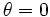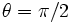# Block suspended from spring along a frictionless inclined plane

(diff) ← Older revision | Latest revision (diff) | Newer revision → (diff)
Jump to: navigation, search
This article discusses a scenario/arrangement whose statics/dynamics/kinematics can be understood using the ideas of classical mechanics.
View other mechanics scenarios

The scenario here is a dry block (with a stable surface of contact) on a dry fixed frictionless inclined plane, with$\theta$ being the angle of inclination with the horizontal axis. In addition, there is a spring with one fixed end and the block attached at the other end, running parallel to the incline, so that if the block slides down the incline, the spring gets stretched and if the block slides up the incline, the spring gets compressed.

We assume that the spring is initially in its relaxed state, so the restoring force on it is zero. Denote by$k$ the spring constant of the spring -- we assume that the spring obeys Hooke's law.

The extremes for the angle$\theta$ are$\theta = 0$ (whence, the plane is horizontal), and$\theta = \pi/2$ (whence, the plane is vertical).

Though the picture depicts a square cross section for the block, this shape assumption is not necessary.

A more general scenario, that considers the possibility of friction along the inclined plane, is discussed at block suspended from spring along an inclined plane.